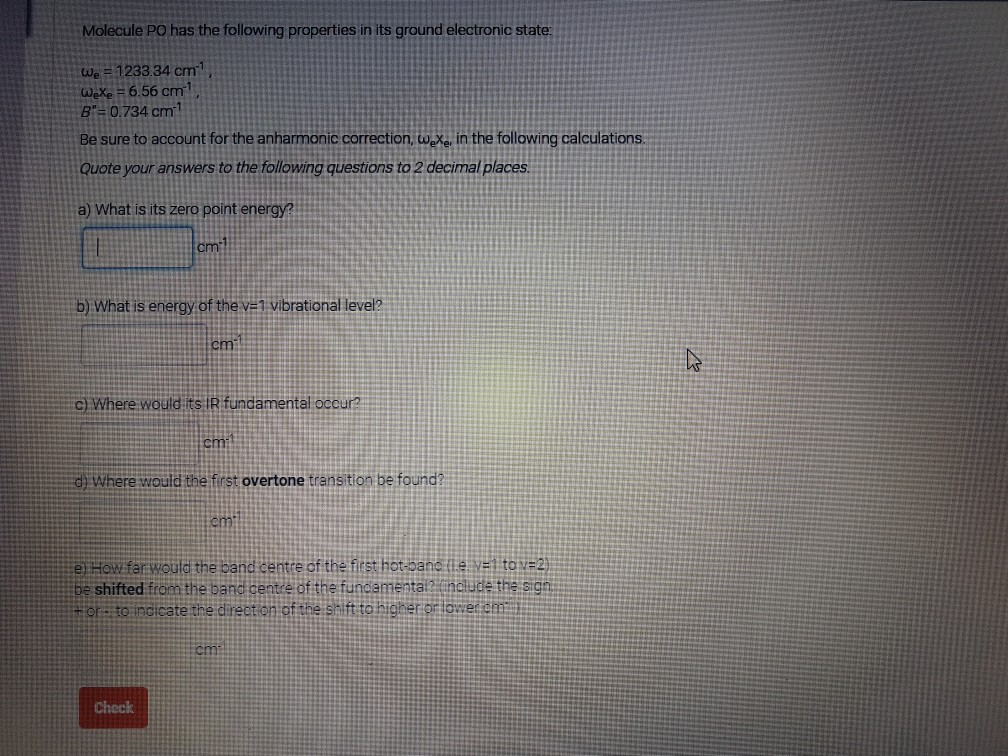# Question & Answer: Molecule PO has the following properties in its ground electronic state: w_e = 1233.34 cm^-1…..Molecule PO has the following properties in its ground electronic state: w_e = 1233.34 cm^-1, w_e x_e = 6.56 cm^-1, B = 0.734 cm^-1 Be sure to account for the anharmonic correction, w_ex_e, in the following calculations. Quote your answer to the following questions to 2 decimal places. a) What is its zero point energy? cm^-1 b) What is energy of the v = 1 vibrational level? cm^-1 c) Where would its IR fundamental occur? cm^-1 d) Where would the first overtone transition be found? cm^-1 e) How far would the band center of the first hot-bane (i.e. v = 1 to v = 2) be shifted from the band center of the fundamental? (Include the sign + or -1 to indicate the direction of the shift to higher or lower cm^2) cm^2

Hooke’s law states that the force tending to restore an atom to its equilibrium position is proportional to the displacement of that from its equilibrium position i.e restoring force

Don't use plagiarized sources. Get Your Custom Essay on
Question & Answer: Molecule PO has the following properties in its ground electronic state: w_e = 1233.34 cm^-1…..
GET AN ESSAY WRITTEN FOR YOU FROM AS LOW AS \$13/PAGE

F= k(r – re) (r-re) – displacement

A system which does not obey Hooke’s law is called an anharmonic oscillator .

P.M . Morse suggested an emperical expression for energy of an anharmonic oscillator

E= Deq[ 1- exp{a(req- r)}]2 Deq – dissociation energy a- constant (req- r)- displacement

Using the Morse energy the Schrodinger eq can solved to cal. the energy of vibrational levels can be given as

Ev = (v+1/2)we – (v+1/2)2wexe we- oscillation frequency xe- anharmonicity const

a. Zero point energy(E0) = 1/2we(1- 1/2xe) = 1/2×1233.34 – 1/4x 6.56 = 615.03 cm-1

b. Ev = (1+1/2)x 1233.34 – (1+ 1/2)26.56= 1835.25 cm-1

c. v= 0 to v= 1   v = +1

E = EV=1 – Ev = 0 = we(1 – 2xe) cm-1 we- IR fundamental

line near we is IR fundamental i.e 1233.34cm-1

d. v= 0 to v =2 , v = +2

E = 2we(1- 3xe)cm-1

line near 2we is first overtone

e. v=1 to v=2 , v = +1   E= we(1- 4xe)cm-1

E= Deq[1- exp{a(req – r )}]2 req – internuclear dist